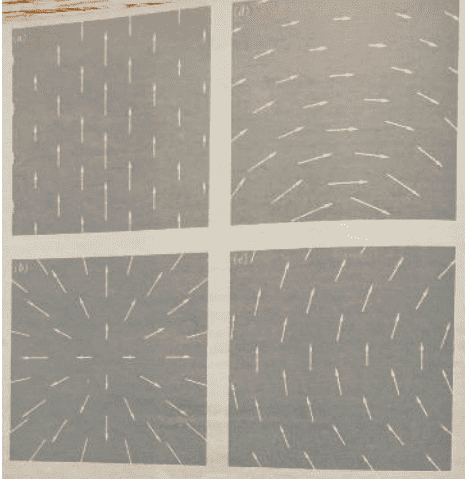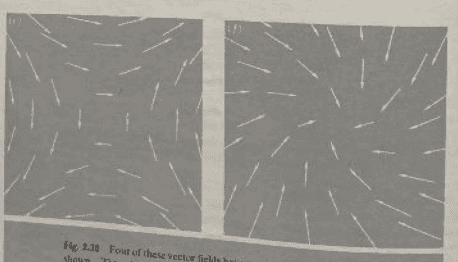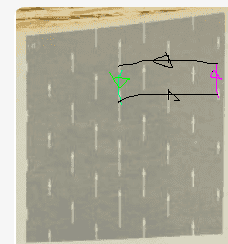# How to observe if a vector field has curl or not?

appletree123
Homework Statement:
So I am supposed to find out if any of the vector fields given has zero curl or not.
Relevant Equations:
So I know that curl has something to do with rotation, and I know how to calculate this vector, but not how to observe if it is zero or not from a picture of the vector field.
These are the vector fields. I really have no idea how to see if there is a curl or not. I have been looking at the rotation of the vector fields. The fields d and e seem to have some rotation or circular paths, but I read online that curl is not about the rotation of the vector field itself, but more about the rotation of points in the vector field. Can someone help me understand how to determine if there is a curl when one are given a picture of a vector field like the ones below?#### Attachments

LCSphysicist
Curl equal zero implies that the path integral of a vector field around some closed path is zero. Of course i am supposing that there are no singularities inside this path, as fas as i remember the name of this condition is to have a simply connected domain.

Anyway, try to follow the reasoning below, for example for the first image:
The arrows more to left have a magnitude lesser than the arrows at the center. Also, both arrows points up. Let's try to follow the path i did at the image:
The arrow path has contribuition ##\int F dr##, the black paths have no contribuition, since they are perpendicular. For the green path, ##\int F' dr'##, where ##|F'|>|F|##, note that the contribuition here is negative.

The total integral is ##\int F dr - F' dr \neq 0##. So curl of F is not zero.•FactChecker
•